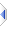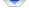# sequence

 Definition: sequence identifier -- or -- bool b = sequence(object x) Description: This serves to define the sequence type. You can also call it like an ordinary function to determine if an object is a sequence. Comments: When invoked as a function, returns true (1) if x is a sequence otherwise false (0). Note that `sequence(x)` and `not atom(x)` are effectively identical. A sequence can hold all string values, but the reverse is not true. Example 1: ```sequence s = {1,2,3} ``` Example 2: ```if sequence(x) then total = 0 for i=1 to length(x) do total += x[i] end for else -- x must be an atom total = x end if ``` Implementation: There are generic versions of the sequence function implemented as :%opSq in builtins\VM\pType.e and :%opSq0 in builtins\VM\pJcc.e that the compiler only invokes as a last resort, preferring instead to inline that functionality if the argument is known to be assigned or the result variable, if any, is known to be an integer, ie does not require decref/dealloc. Note that opSeq is an equality test, and must not be confused with opSq, which is the sequence test. The compiler may also completely omit the test and/or any related code, if type inference/propagation determines that the result will always be true or always false. See Also: atom, integer, string, object, Sequences, Core Language